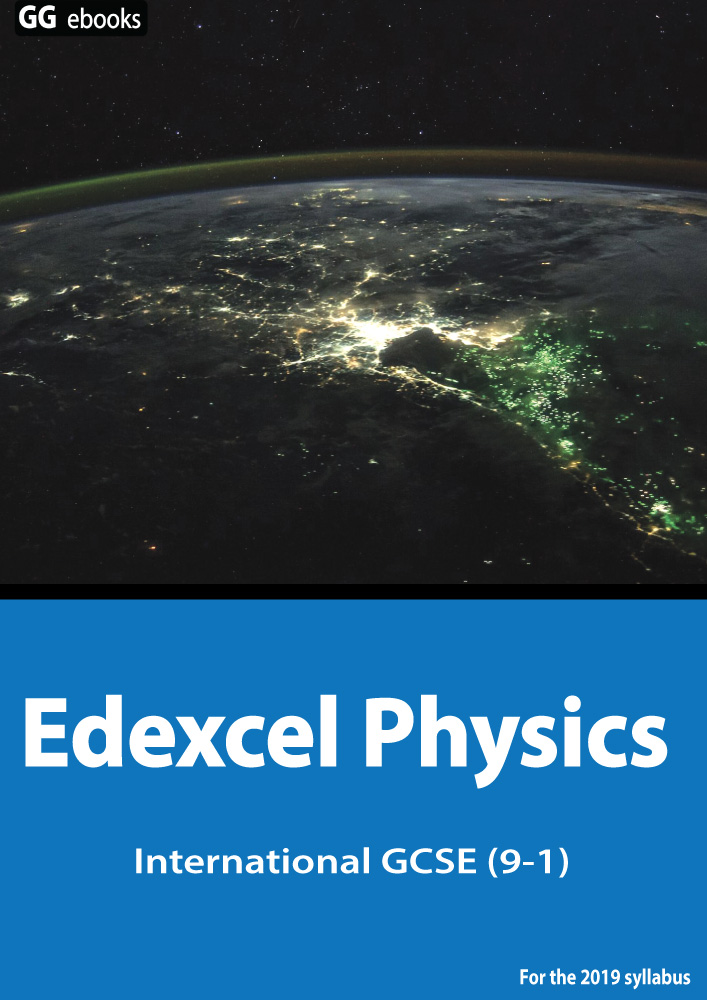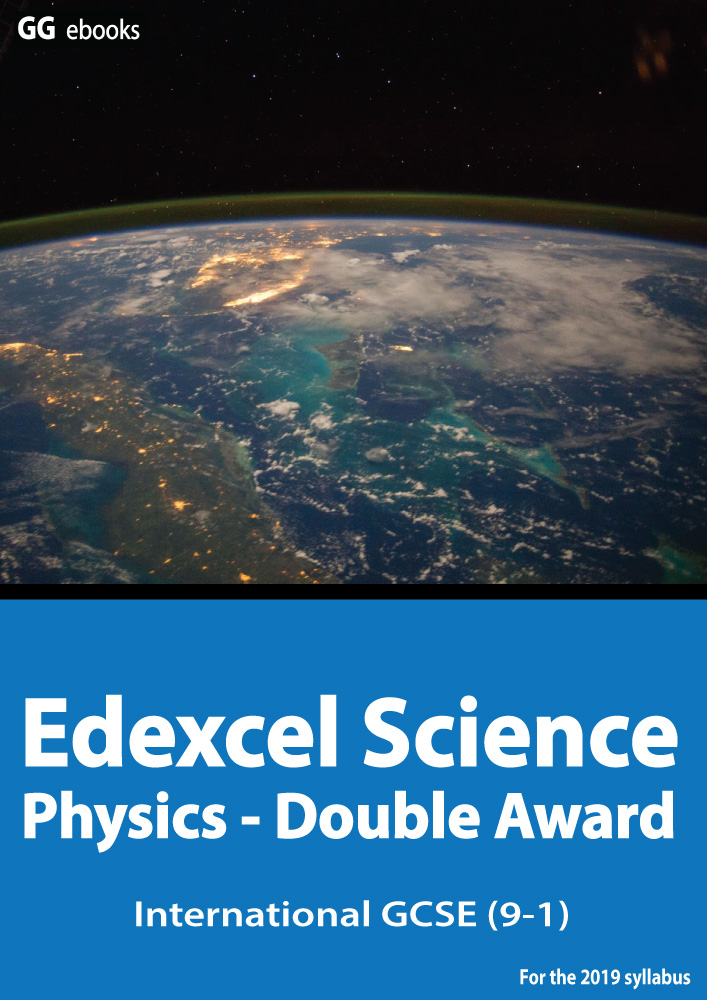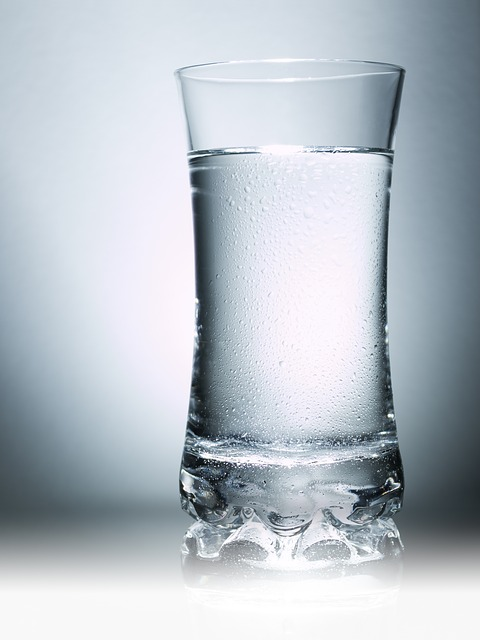µ GCSE | Heat+Matter

10 quick questions - for Edexcel iGCSE

 NEED HELP? Edexcel iGCSE digital textbooks From the makers of GradeGorilla. GGebooks.com (Opens in a new tab)10 minutes maximum! (can you do it in 5?)

Grab a calculator - time for some maths......

Q1-3. The diagram show particles in 3 states of matter:X Y Z

Author:yelod - wikimedia commons - edited

1. Which of these diagrams shows the particles in a gas and a liquid?

• A. 'X' shows a GAS, 'Y' shows a LIQUID.
• B. 'Y' shows a GAS, 'X' shows a LIQUID.
• C. 'Z' shows a GAS, 'X' shows a LIQUID.
• D. 'X' shows a GAS, 'Z' shows a LIQUID.

2. Which of these diagrams shows the particles in their hottest and coldest state?

 Hottest Coldest A Y Z B X Y C Y X D X Z

3. In which of these diagrams are the particles held in place, vibrating about a fixed point?

• A. Y only
• B. Z only
• C. X and Y
• D. Y and Z

The energy needed to heat up a substance is given by the formula shown here:

ΔQ = m c ΔT

change in thermal energy = mass x specific heat capacity x temperature change

4. This formula should be used when a substance is...

• A. melting
• B. boiling
• C. heated but staying in the same state.
• D. staying at the same temperature.

5. Water has a specific heat capacity (c) of 4200 J / Kg 0C.

The energy needed to heat 2 kg of water by 5 0C is therefore:• A. 4200 J
• B. 8400 J
• C. 42000 J
• D. 84000 J

6. If I supply 210 kJ of heat to the same 2 kg of water, how much would the temperature increase?

• A. 25 0C
• B. 50 0C
• C. 100 0C
• D. 250 0C

Q 7-9. These questions are about heating different bars of copper.

Copper has a specific heat capacity of 800 J / Kg 0C.

What are the missing values?Energy Supplied Mass (kg) Temperature Increase (0C) 7. ? 200 J 16 kJ 160 kJ 400 kJ 20 10 8. 8000 J ? 4 10 2 0.5 20 9. 400 kJ 5 ? 4 100 20 200

10. When the ice cubes are first taken out of the freezer they are really cold. Which graph best shows the change in temperature as the ice cubes eventually melt and continue to warm up?